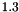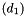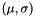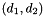## Apophenia

Models

See apop_model for an overview of the intent and basic use of the apop_model struct.

This segment goes into greater detail on the use of existing apop_model objects. If you need to write a new model, see Writing new models.

The estimate function will estimate the parameters of your model. Just prep the data, select a model, and produce an estimate:

apop_data *data = apop_query_to_data("select outcome, in1, in2, in3 from dataset");
apop_model *the_estimate = apop_estimate(data, apop_probit);
apop_model_print(the_estimate);

Along the way to estimating the parameters, most models also find covariance estimates for the parameters, calculate statistics like log likelihood, and so on, which the final print statement will show.

The apop_probit model that ships with Apophenia is unparameterized: apop_probit->parameters==NULL. The output from the estimation, the_estimate, has the same form as apop_probit, but the_estimate->parameters has a meaningful value.

Apophenia ships with many well-known models for your immediate use, including probability distributions, such as the apop_normal, apop_poisson, or apop_beta models. The data is assumed to have been drawn from a given distribution and the question is only what distributional parameters best fit. For example, given that the data is Normally distributed, findandvia apop_estimate(your_data, apop_normal).

There are also linear models like apop_ols, apop_probit, and apop_logit. As in the example, they are on equal footing with the distributions, so nothing keeps you from making random draws from an estimated linear model.

• If you send a data set with the weights vector filled, apop_ols estimates Weighted OLS.
• If the dependent variable has more than two categories, the apop_probit and apop_logit models estimate a multinomial logit or probit.
• There are separate apop_normal and apop_multivariate_normal functions because the parameter formats are slightly different: the univariate Normal keeps bothandin the vector element of the parameters; the multivariate version uses the vector for the vector of means and the matrix for thematrix. The univariate version is so heavily used that it merits a special-case model.

See the Models page for a list of models shipped with Apophenia, including popular favorites like apop_beta, apop_binomial, apop_iv (instrumental variables), apop_kernel_density, apop_loess, apop_lognormal, apop_pmf (see Empirical distributions and PMFs (probability mass functions) below), and apop_poisson.

Simulation models seem to not fit this form, but you will see below that if you can write an objective function for the p method of the model, you can use the above tools. Notably, you can estimate parameters via maximum likelihood and then give confidence intervals around those parameters.

More estimation output

In the apop_model returned by apop_estimate, you will find:

• The actual parameter estimates are in an apop_data set at your_model->parameters.
• A pointer to the apop_data set used for estimation, named data.
• Scalar statistics of the model listed in the output model's info group, which may include some hypothesis tests, a list of expected values, log likelihood, AIC, AIC_c, BIC, et cetera. These can be retrieved via a form like
apop_data_get(your_model->info, .rowname="log likelihood");
//or
apop_data_get(your_model->info, .rowname="AIC");
If those are not necessary, adding to your model an apop_parts_wanted_settings group with its default values (see below on settings groups) signals to the model that you want only the parameters and to not waste possibly significant CPU time on covariances, expected values, et cetera. See the apop_parts_wanted_settings documentation for examples and further refinements.
• In many cases, covariances of the parameters as a page appended to the parameters; retrieve via
apop_data *cov = apop_data_get_page(your_model->parameters, "<Covariance>");
• Typically for regression-type models, the table of expected values (typically including expected value, actual value, and residual) is a page stapled to the main info page. Retrieve via:
apop_data *predict = apop_data_get_page(your_model->info, "<Predicted>");

See individual model documentation for what is provided by any given model.

Post-estimation uses

But we expect much more from a model than estimating parameters from data.

Continuing the above example where we got an estimated Probit model named the_estimate, we can interrogate the estimate in various familiar ways:

apop_data *expected_value = apop_predict(NULL, the_estimate);
double density_under = apop_cdf(expected_value, the_estimate);
apop_data *draws = apop_model_draws(the_estimate, .count=1000);

Data format for regression-type models

# Parameterizing or initializing a model

The models that ship with Apophenia have the requisite procedures for estimation, making draws, and so on, but have parameters==NULL and settings==NULL. The model is thus, for many purposes, incomplete, and you will need to take some action to complete the model. As per the examples to follow, there are several possibilities:

• Estimate it! Almost all models can be sent with a data set as an argument to the apop_estimate function. The input model is unchanged, but the output model has parameters and settings in place.
• If your model has a fixed number of numeric parameters, then you can set them with apop_model_set_parameters.
• If your model has a variable number of parameters, you can directly set the parameters element via apop_data_falloc. For most purposes, you will also need to set the msize1, msize2, vsize, and dsize elements to the size you want. See the example below.
• Some models have disparate, non-numeric settings rather than a simple matrix of parameters. For example, an kernel density estimate needs a model as a kernel and a base data set, which can be set via apop_model_set_settings.

Here is an example that shows the options for parameterizing a model. After each parameterization, 20 draws are made and written to a file named draws-[modelname].

#include <apop.h>
#define print_draws(mm) apop_data_print(apop_model_draws(mm, 20),\
.output_name= "draws-" #mm);
int main(){
apop_model *uniform_20 = apop_model_set_parameters(apop_uniform, 0, 20);
apop_data *d = apop_model_draws(uniform_20, 10);
//Estimate a Normal distribution from the data:
print_draws(N);
//estimate a one-dimensional multivariate Normal from the data:
print_draws(mvN);
//fixed parameter list:
apop_model *std_normal = apop_model_set_parameters(apop_normal, 0, 1);
print_draws(std_normal);
//variable-size parameter list:
std_multinormal->msize1 =
std_multinormal->msize2 =
std_multinormal->vsize =
std_multinormal->dsize = 3;
std_multinormal->parameters = apop_data_falloc((3, 3, 3),
1, 1, 0, 0,
1, 0, 1, 0,
1, 0, 0, 1);
print_draws(std_multinormal);
//estimate a KDE using the defaults:
print_draws(k);
/*A KDE estimation consists of filling an apop_kernel_density_settings group,
so we can set it to use a Normal(μ, 2) kernel via: */
apop_model *k2 = apop_model_set_settings(apop_kernel_density,
.base_data=d,
.kernel = apop_model_set_parameters(apop_normal, 0, 2));
print_draws(k2);
}

# Filtering & updating

The model structure makes it easy to generate new models that are variants of prior models. Bayesian updating, for example, takes in one apop_model that we call the prior, one apop_model that we call a likelihood, and outputs an apop_model that we call the posterior. One can produce complex models using simpler transformations as well. For example, apop_model_fix_params will set the free parameters of an input model to a fixed value, thus producing a model with fewer parameters. To transform a Normal(,) into a one-parameter Normal(, 1):

apop_model *N_sigma1 = apop_model_fix_params(apop_model_set_parameters(apop_normal, NAN, 1));

This can be used anywhere the original Normal distribution can be. To give another example, if we need to truncate the distribution in the data space:

//The constraint function.
double over_zero(apop_data *in, apop_model *m){
return apop_data_get(in) > 0;
}
apop_model *trunc = apop_model_dconstrain(.base_model=N_sigma1,
.constraint=over_zero);

Chaining together simpler transformations is an easy method to produce models of arbitrary detail. In the following example:

• Nature generated data using a mixture of three Poisson distributions, with,, and. The resulting model is generated using apop_model_mixture.
• Not knowing the true distribution, the analyst models the data with a single Poissondistribution with a prior on. The prior selected is a truncated Normal(2, 1), generated by sending the stock apop_normal model to the data-space constraint function apop_dconstrain.
• The apop_update function takes three arguments: the data set, which comes from draws from the mixture, the prior, and the likelihood. It produces an output model which, in this case, is a PMF describing a distribution over, because a truncated Normal and a Poisson are not conjugate distributions. Knowing that it is a PMF, the ->data element holds a set of draws from the posterior.
• The analyst would like to present an approximation to the posterior in a simpler form, and so finds the parametersandof the Normal distribution that is closest to that posterior.

Here is a program—almost a single line of code—that builds the final approximation to the posterior model from the subcomponents, including draws from Nature and the analyst's prior and likelihood:

#include <apop.h>
// For defining the bounds the data-constraining function
// needs to enforce.
double greater_than_zero(apop_data *d, apop_model *m){
return apop_data_get(d) > 0;
}
int main(){
apop_model_set_parameters(apop_poisson, 2.8),
apop_model_set_parameters(apop_poisson, 2.0),
apop_model_set_parameters(apop_poisson, 1.3)
),
1e4
),
apop_model_dconstrain(
.base_model=apop_model_set_parameters(apop_normal, 2, 1),
.constraint=greater_than_zero
),
)->data,
)
);
}

# Model methods

• apop_update : Bayesian updating
• apop_model_coordinate_transform : apply an invertible transformation to the data space
• apop_model_dconstrain : constrain the data space of a model to a subspace. E.g., truncate a Normal distribution so.
• apop_model_fix_params : hold some parameters constant
• apop_model_mixture : a linear combination of models
• apop_model_cross : Ifhas a Normaldistribution andhas an independent Poissondistribution, thenhas an apop_model_cross(apop_normal, apop_poisson) distribution with parameters.

# Settings groups

Describing a statistical, agent-based, social, or physical model in a standardized form is difficult because every model has significantly different settings. An MLE requires a method of search (conjugate gradient, simplex, simulated annealing), and a histogram needs the number of bins to be filled with data.

So, the apop_model includes a single list which can hold an arbitrary number of settings groups, like the search specifications for finding the maximum likelihood, a histogram for making random draws, and options about the model type.

Settings groups are automatically initialized with default values when needed. If the defaults do no harm, then you don't need to think about these settings groups at all.

Here is an example where a settings group is worth tweaking: the apop_parts_wanted_settings group indicates which parts of the auxiliary data you want.

3 apop_model *est = apop_estimate(data, m);

Line one establishes the baseline form of the model. Line two adds a settings group of type apop_parts_wanted_settings to the model. By default other auxiliary items, like the expected values, are set to 'n' when using this group, so this specifies that we want covariance and only covariance. Having stated our preferences, line three does the estimation we want.

Notice that the _settings ending to the settings group's name isn't written—macros make it happen. The remaining arguments to Apop_settings_add_group (if any) follow the Designated initializers syntax of the form .setting=value.

There is an apop_model_copy_set macro that adds a settings group when it is first copied, joining up lines one and two above:

apop_model *m = apop_model_copy_set(apop_ols, apop_parts_wanted, .covariance='y');

Settings groups are copied with the model, which facilitates chaining estimations. Continuing the above example, you could re-estimate to get the predicted values and covariance via:

Apop_settings_set(est, apop_parts_wanted, predicted, 'y');
apop_model *est2 = apop_estimate(data, est);

Maximum likelihood search has many settings that could be modified, and so provides another common example of using settings groups:

apop_model *the_estimate = apop_estimate(data, apop_probit);
//Redo the Probit's MLE search using Newton's Method:
.tolerance=1e-4, .method="Newton");
apop_model *re_est = apop_estimate(data, the_estimate);

To clarify the distinction between parameters and settings, note that parameters are estimated from the data, often via a maximum likelihood search. In an ML search, the method of search, the number of bins in a histogram, or the number of steps in a simulation would be held fixed as the search iterates over possible parameters (and if these settings do change, then that is a meta-model that could be encapsulated into another apop_model). As a consequence, parameters are always numeric, while settings may be any type.

• Apop_settings_set, for modifying a single setting, doesn't use the designated initializers format.
• Because the settings groups are buried within the model, debugging them can be a pain. Here is a documented macro for gdb that will help you pull a settings group out of a model for your inspection, to cut and paste into your .gdbinit. It shouldn't be too difficult to modify this macro for other debuggers.
define get_group
set $group = ($arg1_settings *) apop_settings_get_grp( $arg0, "$arg1", 0 )
p *$group end document get_group Gets a settings group from a model. Give the model name and the name of the group, like get_group my_model apop_mle and I will set a gdb variable named$group that points to that model,
which you can use like any other pointer. For example, print the contents with
p *$group The contents of$group are printed to the screen as visible output to this macro.
end

For using a model, that's all of what you need to know. For details on writing a new settings group, see Writing new settings groups .